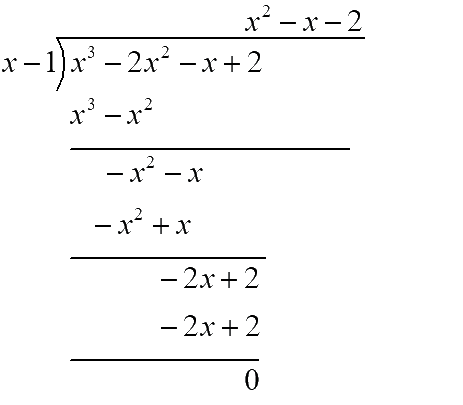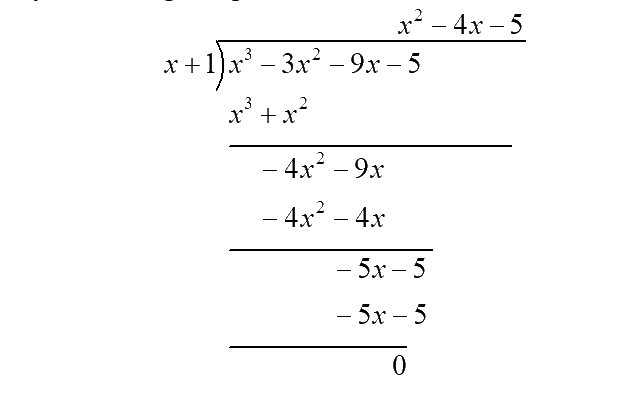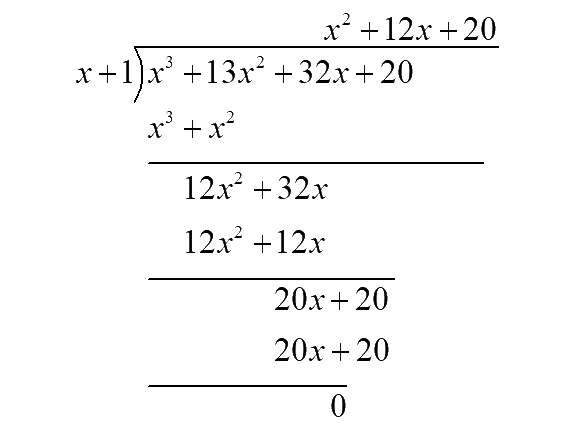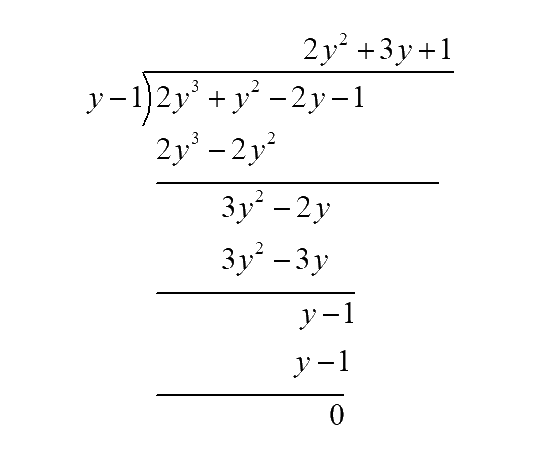# Exercise 2.4 Polynomials NCERT Solutions Class 9

## Chapter 2 Ex.2.4 Question 1

Determine which of the following polynomials has $$(x + 1)$$ a factor:

(i) \begin{align}{x}^{3}+{x}^{2}+x+1\end{align}

(ii) \begin{align}{{x}^{4}}+{{x}^{3}}+{{x}^{2}}+x+1\end{align}

(iii) \begin{align}{{x}^{4}}+3{{x}^{3}}+3{{x}^{2}}+x+1\end{align}

(iv) \begin{align}{{x}^{3}}-{{x}^{2}}-(2+\sqrt{2})x+\sqrt{2}\end{align}

### Solution

Reasoning:

When a polynomial $$p(x)$$ is divided by $$x-a$$ and if $$p(a) = 0$$ then $$(x-a)$$ is a factor of $$p(x)$$. The root of $$x+1=0$$ is $$-1.$$

Steps:

(i) Let \begin{align} p(x)={{x}^{3}}+{{x}^{2}}+x+1\end{align}

\begin{align} \therefore p(-1)&={{(-1)}^{3}}+{{(-1)}^{2}}+(-1)+1 \\ & =-1+1-1+1=0 \\ \end{align}

Since the remainder of $$p\text{(-1) = 0}$$ , we conclude that $$(x+1)$$ is a factor of $${{x}^{3}}+{{x}^{2}}+x+1$$ .

(ii) Let $$p(x)={{x}^{4}}+{{x}^{3}}+{{x}^{2}}+x+1$$

\begin{align}\therefore p( - 1) &\!=\! {( - 1)^4}\!+\!{( - 1)^3}\!+\!{(\!-\!1)^2}\!+\!(\!-\!1)\!+\!1\\ &= \not 1 - \not 1 + \not 1 - \not 1+ 1\\ &= 1 \ne 0\end{align}

Since the remainder of  $$p( - 1) \ne 0$$, we conclude that $$(x+1)$$ in not a factor of  $$\,{x^4} + {x^3} + {x^2} + x + 1$$.

(iii) Let $$p(x) = {x^4} + 3{x^3} + 3{x^2} + x + 1$$

\begin{align} \therefore p( - 1) &\!=\!{( -\!1)^4}\!+\!3{(\!-\!1)^3}\!\!+\!3{(\!-\!1)^2}\!\!+\!( -\!1)\!\!+\!\!1 \\ &= 1 - 3 + 3 - 1 + 1 \\ &= 1 \ne 0\end{align}

Since the remainder of $$p( - 1) \ne 0$$ ,  $$(x+1)$$ is not a factor of $${x^4} + 3{x^3} + 3{x^2} + x + 1$$.

(iv) Let $$p(x) = {x^3} - {x^2} - (2 + \sqrt 2 )x + \sqrt 2$$

\begin{align} \therefore p( - 1) &\!=\!\!{(\!\!-\!1)^3}\!\!-\!{(\!-\!\!1)^2}\!\!-\!(2\!+\!\!\sqrt 2 )( -\!1)\!\!+\!\!\sqrt 2\!\\ &\!=\!-\!1\!-\!1\!+\!2 \!+ \!\sqrt 2\!+\!\sqrt 2\\ &= 2\sqrt 2 \end{align}

Since the remainder of $$p( - 1) = 0$$ , $$(x+1)$$ is  not a factor of $${x^3} - {x^2} - (2 + \sqrt 2 )x + \sqrt 2$$ .

## Chapter 2 Ex.2.4 Question 2

Use the Factor Theorem to determine whether $$g(x)$$ is a factor of $$p(x)$$ in each of the following cases:

(i) $$p(x)\!=\!2{x^3}\!+\!{x^2}\!-\!2x\!-\!1,\,g(x)\!=\!x \!+\!1$$

(ii) $$p(x)\!=\!{x^3}\!+\!3{x^2}\!+\!3x\!+\!1,\,g(x)\!=\!x\!+\!2$$

(iii) $$p(x)\!=\!{x^3}\!-\!4{x^2}\!+\!x\!+\!6,\,g(x)\!=\!x\!-\!3$$

### Solution

Reasoning:

By factor theorem, $$(x-a)$$ is a factor of a polynomial $$p(x)$$ if $$p(a) = 0.$$

To find if $$g(x )= x+a$$ is a factor of $$p(x),$$ we need to find the root of $$g(x).$$

$$x + a = 0{\rm{ }} \to {\rm{ }}x{\rm{ }} = -a$$

Steps:

(i) Let $$p(x) = 2{x^3} + {x^2} - 2x - 1,\,g(x) = x + 1$$

$x + 1 = 0{\rm{ }} \to {\rm{ }}x{\rm{ }} = {\rm{ }}-1$

Now,

\begin{align}p( - 1) &= 2{( - 1)^3} + {( - 1)^2} - 2( - 1) - 1\\ & = - \not 2 + \not 1 + \not 2 - \not 1\\& = 0\end{align}

Since the remainder of $$p( - 1) = 0$$ , by factor theorem we can say $$g(x) = x+1$$ is a factor of $$p(x) = 2{x^3} + {x^2} - 2x - 1.$$

(ii) Let $$p(x) = {x^3} + 3{x^2} + 3x + 1,\,\,g(x) = x + 2$$

$x + 2 = 0{\rm{ }} \to {\rm{ }}x{\rm{ }} = {\rm{ }}-2$

Now,

\begin{align}p( - 2)&= {( - 2)^3} + 3{( - 2)^2} + 3( - 2) + 1\\ & = - 8 + 12 - 6 + 1\\ & = - 1 \ne 0\end{align}

Since the remainder of $$p( - 2) \ne 0$$ , by factor theorem we can say $$g(x) = x+2$$ is not a factor of $$p(x) = {x^3} + 3{x^2} + 3x + 1.$$

(iii) Let $$p(x) = {x^3} - 4{x^2} + x + 6,\,\,g(x) = x - 3$$

$x - 3 = 0{\rm{ }} \to {\rm{ }}x\,{\rm{ = }}\,{\rm{3}}$

Now,

\begin{align}p(3) &= {(3)^3} - 4{(3)^2} + 3 + 6\\ & = 27 - 36 + 3 + 6\\ & = 0\end{align}

Since the remainder of $$p(3) = 0$$ , by factor theorem we can say $$g(x) = x-3$$ is a factor of $$p(x) = {x^3} - 4{x^2} + x + 6.$$

## Chapter 2 Ex.2.4 Question 3

Find the value of $$k,$$ if $$x – 1$$ is a factor of $$p(x)$$ in each of the following cases:

### Solution

(i) \begin{align} p(x) = {x^2} + x + k\end{align}

(ii) \begin{align} p(x) = 2{x^2} + kx + \sqrt 2 \end{align}

(iii)  \begin{align} p(x) = k{x^2} - \sqrt {2x} + 1 \end{align}

(iv)  \begin{align} p(x) = k{x^2} - 3x + k\end{align}

Reasoning:

By factor theorem, if $$x-1$$ is a factor of $$p(x),$$ then $$p\left( 1 \right) = 0$$.

Steps:

(i) \begin{align} p(x) = {x^2} + x + k\end{align}

\begin{align}\,\,\,\, p(x) &= {x^2} + x + k\\ p(1) &= {(1)^2} + (1) + k\\ \,\,\,\,\,\,\,0\,\, &= 2 + k\\ \,\,\,\,\,\,\,\,\,\,\,\,\,\,\,\, \Rightarrow k &= - 2\end{align}

(ii) \begin{align} p(x) = 2{x^2} + kx + \sqrt 2 \end{align}

\begin{align}\,\,\,\, p(x) &= 2{x^2} + kx + \sqrt 2 \\ p(1) &= 2{(1)^2} + k(1) + \sqrt 2 \\ \,\,\,\,\,\,\,0\,\, &= 2 + k + \sqrt 2 \\ \,\,\,\,\,\,\,\,\,\,\,\,\,\,\,\, \Rightarrow k &= - (2 + \sqrt 2 )\end{align}

(iii)  \begin{align} p(x) = k{x^2} - \sqrt {2x} + 1 \end{align}

\begin{align}\,\,\,\,\,\,\,\, p(x) &= k{x^2} - \sqrt {2x} + 1\\ p(1) &= k{(1)^2} - \sqrt {2(1)} + 1\\ \,\,\,\,\,\,\,\,0 &= k - \sqrt 2 + 1\\ \,\,\,\,\,\,\,\,\,\,\,\,\,\,\,\, \Rightarrow k &= \sqrt 2 - 1\end{align}

(iv)  \begin{align} p(x) = k{x^2} - 3x + k\end{align}

\begin{align}\,\,\, p(x) &= k{x^2} - 3x - k\\ p(1) &= k({1^2}) - 3(1) - k\\ \,\,\,\,\,\,\,\,0 &= 2k - 3\\\,\,\,\,\,\,\,\,\,\,\,\,\,\,\,\, \Rightarrow k &= \frac{3}{2}\end{align}

## Chapter 2 Ex.2.4 Question 4

Factorise:

(i)\begin{align}12{x^2} - 7x + 1 \end{align}

(ii)\begin{align}2{x^2} + 7x + 3 \end{align}

(iii) \begin{align}6{x^2} + 5x - 6 \end{align}

(iv) \begin{align}3{x^2} - x - 4 \end{align}

### Solution

Reasoning:

By splitting method, we can find factors using the following method.

Find $$2$$ numbers $$p, q$$ such that:

(i) $$p + q =$$ co-efficient of $$x$$

(ii) $$pq =$$ co-efficient of $${x^2}$$ and the constant term.

Steps:

(i) $$\,12{x^2} - 7x + 1$$

$$p + q = - 7$$(co-efficient of $$x$$)

$$pq = 12 \times 1 = 12$$ (co-efficient of $${x^2}\,$$ and the constant term.)

By trial and error method, we get $$p = -4, q = -3.$$

Now splitting the middle term of the given polynomial,

\begin{align}12{x^2} - 7x + 1 &= 12{x^2} - 4x - 3x + 1\\ &= 4x(3x - 1) - 1(3x - 1)\\ &= (3x - 1)(4x - 1) \\&\text {(taking} (3x\!-\!1\!)\!\text{ as common)}\end{align}

(ii) $$\,\,2{x^2} + 7x + 3$$

$$p + q = 7$$ (co-efficient of $$x$$)

$$pq = 2 \times 3 = 6$$ (co-efficient of $${x^2}\,$$ and the constant term.)

By trial and error method, we get $$p = 6, q = 1.$$

Now splitting the middle term of the given polynomial,

\begin{align}\,2{x^2} + 7x + 3 &= 2{x^2} + 6x + x + 3\\\,\,\,\,\,\,\,\,\,\,\,\,\,\,\,\,\,\,\,\,\,\,\,\,\,\,\,\,\,\, &= 2x(x + 3) + 1(x + 3)\\ \,\,\,\,\,\,\,\,\,\,\,\,\,\,\,\,\,\,\,\,\,\,\,\,\,\,\,\,\,\,\,\, &= (2x + 1)(x + 3)\end{align}

(iii) $$6{x^2} + 5x - 6$$

$$p + q = 5$$ (co-efficient of $$x$$)

$$pq = 6 \times -6 = 36$$ (co-efficient of $${x^2}\,$$ and the constant term.)

By trial and error method, we get $$p = 9, q = -4.$$

Now splitting the middle term of the given polynomial,

\begin{align} 6{x^2} + 5x - 6 &= 6{x^2} + 9x - 4x - 6\\ \,\,\,\,\,\,\,\,\,\,\,\,\,\,\,\,\,\,\,\,\,\,\,\,\,\,\,\,\,\, &= 3x(2x + 3) - 2(2x + 3)\\ \,\,\,\,\,\,\,\,\,\,\,\,\,\,\,\,\,\,\,\,\,\,\,\,\,\,\,\,\,\, &= (3x - 2)(2x + 3)\end{align}

(iv) $$3{x^2} - x - 4$$

$$p + q = -1$$ (co-efficient of $$x$$)

$$pq = 3 \times -4 = -12$$(co-efficient of $${x^2}\,$$ and the constant term.)

By trial and error method, we get $$p = -4, q = 3.$$

Now splitting the middle term of the given polynomial,

\begin{align} 3{x^2} - x - 4 &= 3{x^2} - 4x + 3x - 4\\ \,\,\,\,\,\,\,\,\,\,\,\,\,\,\,\,\,\,\,\,\,\,\,\,\,\, &= \,\,3{x^2} + 3x - 4x - 4\\ \,\,\,\,\,\,\,\,\,\,\,\,\,\,\,\,\,\,\,\,\,\,\,\,\,\, &= \,3x(x + 1) - 4(x + 1)\\ \,\,\,\,\,\,\,\,\,\,\,\,\,\,\,\,\,\,\,\,\,\,\,\,\,\, &= (3x - 4)(x + 1)\end{align}

## Chapter 2 Ex.2.4 Question 5

Factorise:

(i) \begin{align}{x^3} - 2{x^2} - x + 2 \end{align}

(ii) \begin{align}{x^3} - 3{x^2} - 9x - 5\end{align}

(iii) \begin{align}{x^3} + 13{x^2} + 32x + 20 \end{align}

(iv) \begin{align}2{y^3} + {y^2} - 2y - 1\end{align}

### Solution

Steps:

(i) Let $$p(x) = {x^3} - 2{x^2} - x + 2$$

By the factor theorem we know that $$x-a$$ is a factor of $$p(x)$$if $$p(a) = 0.$$

We shall find a factor of $$p(x)$$ by using some trial value of $$x,$$ say $$x = 1.$$

\begin{align} p(1) &= {(1)^3} - 2{(1)^2} - 1 + 2\\ &= 1 - 2 - 1 + 2 = 0\end{align}

Since the remainder of $$p(1) = 0$$ , by factor theorem we can say $$x=1$$ is a factor of $$p(x) = {x^3} - 2{x^2} - x + 2.$$

Now divide $$p(x)$$ by $$x-1$$ using long division,Hence $${x^3}\!-\!\! 2{x^2}\! -\! x\!+\!\!2\!=\!(x\!-\!\!1)({x^2}\!-\!x\!-\!\!2)$$

Now taking $${x^2} - x - 2$$ , find $$2$$ numbers $$p, q$$ such that:

(i) $$p + q =$$co-efficient of $$x$$

(ii) $$pq =$$ co-efficient of $${x^2}$$ and the constant term.

$$p + q = - 1$$ (co-efficient of $$x$$)

$$pq = 1 \times - 2 = - 2$$(co-efficient of $${x^2}$$ and the constant term.)

By trial and error method, we get $$p = -2, q = 1.$$

Now splitting the middle term of the given polynomial,

\begin{align} {x^2} - x - 2 &= {x^2} - 2x + x - 2\\ \,\,\,\,\,\,\,\,\,\,\,\,\,\,\,\,\,\,\,\,\,\,\,\, &= x(x - 2) + 1(x - 2)\\ \,\,\,\,\,\,\,\,\,\,\,\,\,\,\,\,\,\,\,\,\,\,\,\, &= (x + 1)(x - 2)\end{align}

$$\therefore\!{x^3}\!\!- \!2{x^2}\!\!-\!x\!+\!2\!=\!(x\!\!-\!1)\!(x\!-\!2)(x\!+\!\!1)$$

Method 2:

\begin{align}{x^3}\!\!-\!2{x^2}\!\!-\!x\!+\!2\!&=\!({x^3}\!\!-\!2{x^2})\!-\!(x\!\!-\!\!2)\\ &= {x^2}(x - 2)\!-\!1(x\! -\!\! 2)\\ &= (x - 2)({x^2} - 1)\\ &= (x\!-\!2)(x\!+\!1)(x\!-\!\! 1) \\&\left( \begin{array}{l}{\text{By using }}{a^2} - {b^2}\\ = (a + b)(a - b)\end{array} \right)\end{align}

(ii) Let $$p(x) = {x^3} - 3{x^2} - 9x - 5$$

By the factor theorem we know that $$x-a$$ is a factor of $$p(x)$$ if $$p(a) = 0.$$

We shall find a factor of $$p(x)$$ by using some trial value of $$x,$$ say $$x = 1.$$

\begin{align}p(1) &= {(1)^3} - 3{(1)^2} - 9(1) - 5 \\ &= 1 - 3 - 9 - 5\\ &= - 16 \ne 0\end{align}

Since the remainder of $$p(1) \ne 0$$ , by factor theorem we can say $$x=1$$ is not a factor of $$p(x) = {x^3} - 3{x^2} - 9x - 5.$$

Now say $$x = -1.$$

\begin{align} p(\!-\!1) &\!=\!\!{(\!-\!1)^3}\!-\!\!3{( -\!1)^2}\!\!-\!\! 9(\!-\!1)\!-\!\!5 \\ &= - 1 - 3 + 9 - 5\\ &= - 9 + 9 = 0\end{align}

Since the remainder of $$p( - 1) = 0$$ , by factor theorem we can say $$x=-1$$ is a factor of $$p(x) = {x^3} - 3{x^2} - 9x - 5.$$

Now dividing $$p(x)$$ by $$x+1$$using long division.Hence $${x^3} -\!\!3{x^2}\!\!-\!\!9x\!-\!\!5\!\!=\!\!(x\!+\!1)\!({x^2}\!-\!4x\!-\!5)$$

Now taking $${x^2} - 4x - 5$$ , find $$2$$ numbers $$p, q$$ such that:

(i) $$p + q =$$ co-efficient of $$x$$

(ii) $$pq =$$ co-efficient of $${x^2}$$ and the constant term.

$$p + q = - 4$$ (co-efficient of $$x$$)

$$pq = 1 \times - 5 = - 5$$ (co-efficient of $${x^2}$$ and the constant term.)

By trial and error method, we get $$p = -5, q = 1.$$

Now splitting the middle term of the given polynomial,

\begin{align} {x^2} - 4x - 5 &= {x^2} - 5x + x - 5\\ \,\,\,\,\,\,\,\,\,\,\,\,\,\,\,\,\,\,\,\,\,\,\,\, &= x(x - 5) + 1(x - 5)\\ \,\,\,\,\,\,\,\,\,\,\,\,\,\,\,\,\,\,\,\,\,\,\,\, &= (x + 1)(x - 5) \end{align}

\begin{align}&\therefore {x^3}\!-\!\!2{x^2}\!-\!\!x\!+\!2 \\&= (x\!+\!1)(x\!-\!5)(x\!+\!1)\\ &= \,{(x\!+\!1)^2}\!(x\!- \!\!5)\,\, \end{align}

(iii) Let $$p(x) = {x^3} + 13{x^2} + 32x + 20$$

By the factor theorem we know that $$x-a$$ is a factor of $$p(x)$$ if $$p(a) = 0.$$

We shall find a factor of $$p(x)$$ by using some trial value of $$x,$$ say $$x = -1.$$ (Since all the terms are positive.)

\begin{align}\!p(\!-\!1) &\!=\!{(\!- 1)^3}\!+\!\!13{( - 1)^2}\!\!+\!32( - 1)\!+\!\!20 \\ &= - 1 + 13 - 32 + 20\\ &= 0 \end{align}

Since the remainder of $$p( - 1) = 0$$ , by factor theorem we can say $$x= -1$$ is a factor of $$p(x) = {x^3} + 13{x^2} + 32x + 20.$$

Now dividing $$p(x)$$ by $$x+1$$ using long division,$\left[ \begin{array}{l}\therefore {x^3} + 13{x^2} + 32x + 20\\ = \,(x + 1)({x^2} + 12x + 20)\end{array} \right]$

Now taking $${x^2} + 12x + 20$$ , find $$2$$ numbers $$p, q$$ such that:

(i) $$p + q =$$ co-efficient of $$x$$

(ii) $$pq =$$ co-efficient of $${x^2}$$ and the constant term.

$$p + q = 12$$ (co-efficient of $$x$$)

$$pq = 1 \times 20 = 20$$ (co-efficient of $${x^2}$$ and the constant term.)

By trial and error method, we get $$p = 10, q = 2.$$

Now splitting the middle term of the given polynomial,

\begin{align} {x^2}\!\!+\!12x\!+\!20\!&=\!{x^2}\!\!+\!10x\!+\!\!2x\!+\!\!20\\ &=\!x(x\!+\!\!10)\!+\!\!2(x\!+\!\!10)\\ &= (x\!+\!\!10)\!(x\!+\!\!2)\end{align}

$\left( \begin{array}{l}\therefore {x^3} + \,13{x^2} + \,32x\, + \,20\\ = (x + 1)\,(x + 10)\,(x + 2)\end{array} \right)$

Method 2:

\begin{align}&{x^3} + 13{x^2} + 32x + 20 \\&=\!{x^3}\!+\!10{x^2}\!+\!3{x^2}\!+\!30x\!+\!2x\!+\!20\\ &= {x^2}\!(x\!+\!\!10)\!+\!\!3x(x\!+\!10)\!+\!\!2(\!x\!+\!10\!) \\ &= (x + 10)({x^2} + 3x + 2)\\ &= (x + 10)({x^2} + 2x + x + 2) \\ &= (x + 10)[x(x + 2) + 1(x + 2)]\\ &= (x + 10)(x + 2)(x + 1)\end{align}

(iv) Let  $$p(y) = 2{y^3} + {y^2} - 2y - 1$$

By the factor theorem we know that $$(y-a)$$ is a factor of $$p(y)$$ if $$p(a) = 0.$$

We shall find a factor of $$p(y)$$ by using some trial value of $$y,$$ say $$y = 1.$$

\begin{align} p(1) &= 2{(1)^3} + {(1)^2} - 2(1) - 1\\ \,\,\,\,\,\,\,\,\,\,\,\, &= 2 + 1 - 2 - 1\\ \,\,\,\,\,\,\,\,\,\,\,\, &= 0 \end{align}

Since the remainder of $$p(1) = 0$$ , by factor theorem we can say $$y-1$$ is a factor of $$p(y) = 2{y^3} + {y^2} - 2y - 1$$

Now dividing $$p(y)$$ by $$y-1$$ using long division,$$\therefore\!2{y^3}\!+\!{y^2}\!-\!2y\!-\!\!1\!\!=\!\!(y\!\!-\!\!1)\!(2{y^2}\!+\!3y\!+\!1)$$

Now taking $$2{y^2} + 3y + 1$$ , find $$2$$ numbers $$p, q$$ such that:

(i) $$p + q =$$co-efficient of $$y$$

(ii) $$pq =$$ co-efficient of $${y^2}$$ and the constant term.

$$p + q = 3$$ (co-efficient of $$y$$)

$$pq = 2 \times 1 = 2$$(co-efficient of $${y^2}$$ and the constant term.)

By trial and error method, we get $$p = 2, q = 1.$$

Now splitting the middle term of the given polynomial,

\begin{align} 2{y^2} + 3y + 1 &= 2{y^2} + 2y + y + 1 \\ &= 2y(y + 1) + 1(y + 1)\\&= (2y + 1)(y + 1)\end{align}

$$\!\therefore\!2{y^3}\!+\!\!{y^2}\!-\!2y\!-\!\!1\!\!=\!\!(y\!-\!1)(2y\!+\!\!1)\!(y\!+\!\!1)$$

Instant doubt clearing with Cuemath Advanced Math Program